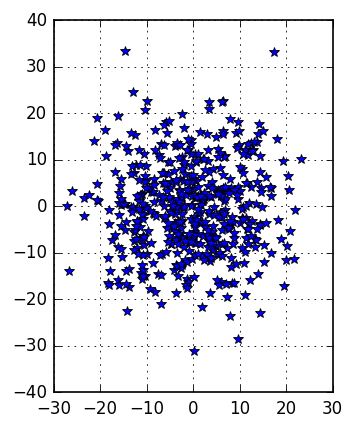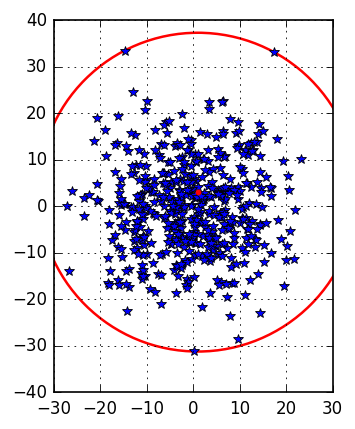# Smallest sphere enclosing a set of points.¶

The aim of this tutorial is two-fold

1. Demostrate how to write a conic quadratic model in Fusion in a very simple and compact way.
2. Show how and way the dual formulation may solved more efficiently.

Our problem is the defined as:

Find the smallest sphere that encloses a set of $k$ points $p_i \in \mathbb{R}^n$.

In :
%matplotlib inline
import matplotlib.pyplot as plt
import matplotlib.patches as mpatches
import random

def plot_points(p, p0=[], r0=0.):
n,k= len(p0), len(p)

plt.rc('savefig',dpi=120)

fig, ax = plt.subplots()
ax.set_aspect('equal')
ax.plot([ p[i] for i in range(k)], [ p[i] for i in range(k)], 'b*')

if len(p0)>0:
ax.plot(  p0,p0, 'r.')
ax.add_patch( mpatches.Circle( p0,  r0 ,  fc="w", ec="r", lw=1.5) )
plt.grid()
plt.show()

n = 2
k = 500

p=  [ [random.gauss(0.,10.) for nn in range(n)] for kk in range(k)]

plot_points(p)The problem boils down to determine the sphere center $p_0\in \mathbb{R}^n$ and its radius $r_0\geq 0$, i.e.

\begin{equation} \begin{aligned} \min \max_{i=1,\dots,k} \| p_0 - p_i\|_2 \\ \end{aligned} \end{equation}

The maximum in the objective function can be easily, i.e.

\begin{equation} \begin{aligned} \min r_0 & & &\\ s.t.& & &\\ & r_0 \geq \| p_0 - p_i\|_2 ,& \quad & i=1,\ldots,k\\ \end{aligned} \end{equation}

\begin{equation} \begin{aligned} \min r_0 & & &\\ s.t.& & &\\ & \left[r_0,p_0 - p_i\right] \in Q^{(n+1)}, & \quad & i=1,\ldots,k. \end{aligned} \end{equation}

Before defining the constraints, we note that we can write

\begin{equation} R_0 = \left( \begin{array}{c} r_0 \\ \vdots \\ r_0 \end{array} \right) \in \mathbb{R}^k , \quad P_0 = \left( \begin{array}{c} p_0^T \\ \vdots \\ p_0^T \end{array} \right) \in \mathbb{R}^{k\times n}, \quad P = \left( \begin{array}{c} p_1^T \\ \vdots \\ p_k^T \end{array} \right) \in \mathbb{R}^{k\times n}. \end{equation}

so that

\begin{equation} \left[r_0,p_i - p_0\right] \in Q^{(n+1)}, \quad i=1,\ldots,k. \end{equation}

can be compactly expressed as

\begin{equation} \left[ R_0,P_0-P\right] \in \Pi Q^{(n+1)}, \end{equation}

that means, with a little abuse of notation, that each rows belongs to a quadratic cone of dimension $n+1$.

Now we are ready for a compact implementation in Fusion:

In :
from mosek.fusion import *
import mosek as msk

def primal_problem(P):

print(msk.Env.getversion())

k= len(P)
if k==0: return -1,[]

n= len(P)

with Model("minimal sphere enclosing a set of points - primal") as M:

r0 = M.variable(1    , Domain.greaterThan(0.))
p0 = M.variable([1,n], Domain.unbounded())

R0 = Var.repeat(r0,k)
P0 = Var.repeat(p0,k)

M.constraint( Expr.hstack( R0, Expr.sub(P0 , P) ), Domain.inQCone())

M.objective(ObjectiveSense.Minimize, r0)
M.setLogHandler(open('logp','wt'))

M.solve()

return r0.level(), p0.level()



We will also store the solver output in a file to use it later on. And then just solve the problem.

In :
r0,p0 = primal_problem(p)

print ("r0^* = ", r0)
print ("p0^* = ", p0)

(8, 0, 0, 48)
('r0^* = ', 34.277010059679036)
('p0^* = ', array([ 0.9775514 ,  3.05181728]))

In :
plot_points(p,p0,r0)## Dual Formulation ¶

The dual problem can be determined in few steps following basic rules. Introducing dual variables

\begin{equation} Y = \left( \begin{array}{c} y_1^T\\ \vdots \\y_k \end{array}\right), \quad z = \left( \begin{array}{c} z_1\\ \vdots \\z_k \end{array}\right), \end{equation}

the dual is:

\begin{aligned} \max & \left\langle P, Y \right\rangle \\ & e_k^T z = 1\\ & Y^T e_k = \mathbf{0}_n \\ & \left[z_i , y_i\right] \in \mathcal{Q}^{n+1}\\ & z_i\in \mathbb{R}, y_i\in \mathbb{R}^n, \end{aligned}

where $e_k\in \mathbb{R}^k$ is a vector of all ones.

The Fusion code is the following:

In :
def dual_problem(P):

k= len(P)
if k==0: return -1,[]

n= len(P)

with Model("minimal sphere enclosing a set of points - dual") as M:

Y= M.variable([k,n], Domain.unbounded())
z= M.variable(k    , Domain.unbounded())

M.constraint(Expr.sum(z), Domain.equalsTo(1.) )

e= [1.0 for i in range(k)]

M.constraint(Expr.mul(Y.transpose(), Matrix.ones(k,1)), Domain.equalsTo(0.) )

M.constraint( Var.hstack(z,Y), Domain.inQCone())

M.objective( ObjectiveSense.Maximize, Expr.dot( P, Y ))

M.setLogHandler(open('logd','wt'))

M.solve()

return

dual_problem(p)


Looking at the solver output for the primal and dual implementation we get

In :
!tail  logp

11  4.7e-06  2.8e-04  1.9e-05  1.01e+00   3.429062907e+01   3.430435587e+01   5.6e-07  0.01
12  1.3e-06  7.5e-05  1.1e-05  1.00e+00   3.427957004e+01   3.428275209e+01   1.5e-07  0.01
13  2.2e-07  1.3e-05  4.8e-06  1.00e+00   3.427737473e+01   3.427786148e+01   2.7e-08  0.01
14  2.1e-08  1.2e-06  1.6e-06  1.00e+00   3.427703453e+01   3.427707456e+01   2.5e-09  0.01
15  2.8e-09  1.7e-07  5.7e-07  1.00e+00   3.427701143e+01   3.427701671e+01   3.3e-10  0.01
16  5.3e-10  3.2e-08  2.7e-07  1.00e+00   3.427701016e+01   3.427701104e+01   6.3e-11  0.01
17  3.1e-11  1.4e-09  4.5e-08  1.00e+00   3.427701006e+01   3.427701009e+01   2.7e-12  0.01
Interior-point optimizer terminated. Time: 0.01.

Optimizer terminated. Time: 0.10

In :
!tail logd

11  2.8e-04  4.7e-06  1.9e-05  1.01e+00   3.430435567e+01   3.429062880e+01   5.6e-07  0.01
12  7.5e-05  1.3e-06  1.1e-05  1.00e+00   3.428275207e+01   3.427956999e+01   1.5e-07  0.01
13  1.3e-05  2.2e-07  4.8e-06  1.00e+00   3.427786147e+01   3.427737472e+01   2.7e-08  0.01
14  1.2e-06  2.1e-08  1.6e-06  1.00e+00   3.427707456e+01   3.427703453e+01   2.5e-09  0.01
15  1.7e-07  2.8e-09  5.7e-07  1.00e+00   3.427701671e+01   3.427701143e+01   3.3e-10  0.01
16  3.2e-08  5.3e-10  2.7e-07  1.00e+00   3.427701104e+01   3.427701016e+01   6.3e-11  0.01
17  1.4e-09  2.7e-11  5.8e-08  1.00e+00   3.427701009e+01   3.427701006e+01   2.7e-12  0.01
Interior-point optimizer terminated. Time: 0.01.

Optimizer terminated. Time: 0.01


The solver has got the same result doing exacly the same steps!

In fact, MOSEK has solve the same problem, thanks to the automatic dualizer that decides whether is more convenient to solve the primal or the dual of a given problem. To see that, just look at the output again:

In :
!grep Optimizer logp

Optimizer started.
Optimizer  - solved problem         : the dual
Optimizer  - Constraints            : 3
Optimizer  - Cones                  : 500
Optimizer  - Scalar variables       : 1501              conic                  : 1500
Optimizer  - Semi-definite variables: 0                 scalarized             : 0
Optimizer terminated. Time: 0.10

In :
!grep Optimizer logd

Optimizer started.
Optimizer  - solved problem         : the primal
Optimizer  - Constraints            : 3
Optimizer  - Cones                  : 500
Optimizer  - Scalar variables       : 1500              conic                  : 1500
Optimizer  - Semi-definite variables: 0                 scalarized             : 0
Optimizer terminated. Time: 0.01


In primal problem output the solver reports it is going to solve the dual, i.e. our second formulation. In the dual problem output it says it is going to solve the primal. Therefore in both cases we actually solve the very same formulation.This work is licensed under a Creative Commons Attribution 4.0 International License. The MOSEK logo and name are trademarks of Mosek ApS. The code is provided as-is. Compatibility with future release of MOSEK or the Fusion API are not guaranteed. For more information contact our support.

In [ ]: